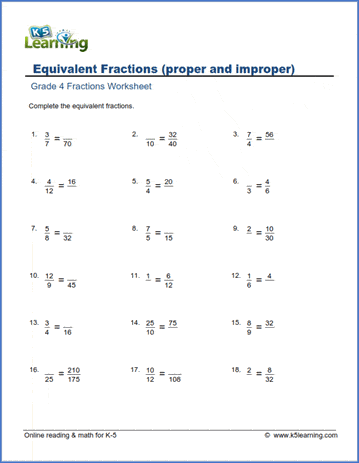# Equivalent fractions (proper & improper)

## Worksheets: Equivalent fractions (proper and improper fractions)

Below are six versions of our grade 4 fractions worksheet on equivalent fractions. Students must fill in the missing numerator or denominator to make the two fractions shown equivalent.  Fractions may be proper or improper fractions. These worksheets are pdf files.## More fractions worksheets

Explore all of our fractions worksheets, from dividing shapes into "equal parts" to multiplying and dividing improper fractions and mixed numbers.

## What is K5?

K5 Learning offers free worksheets and inexpensive workbooks for kids in kindergarten to grade 5.  We help your children build good study habits and excel in school.